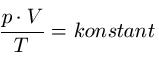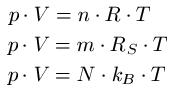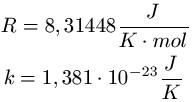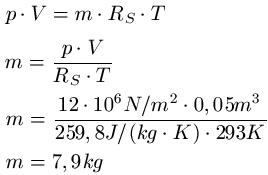# What are gas laws

## Gas laws

We deal with the gas law or the gas laws in this article. We limit ourselves to the calculation of ideal gases and provide a corresponding example. This article belongs to the field of thermodynamics.The gas laws are used to describe ideal gases (reading tip: ideal gas). The formulas for the gas laws provide a relationship between the variables pressure, volume, amount of substance and the absolute temperature. Since the amount of substance is given in moles, another reading tip from the field of chemistry: Mol / Molar mass.

Regarding the gas law: Under the condition of a closed gas quantity, the following relationship exists for the ideal gas:There are a number of other formulas for calculating the relationships that we would now like to introduce to you. Below the formulas you will find information about what the respective variables stand for and an application example.

Gas laws formulas:Meaning of the variables:

• "p" is the pressure in Pascals or Newtons per square meter [Pa or N / m2 ]
• "V" is the volume in cubic meters [m3 ]
• "n" is the amount of substance in mol [mol]
• "R" is the universal gas constant in Joule per Kelvin times Mol [J / Kmol]
• "T" is the temperature in Kelvin [K]
• "m" is the mass of the gas in kilograms [kg]
• "RS."is the specific gas constant in joules per Kelvin times kilogram [J / (kg · K)]
• "N" corresponds to the number of particles, without a unit
• "kB."or" k "is the Boltzmann constant in joules per Kelvin [J / K]

Constant values:Example:

For a better understanding an example of the gas laws: In a vessel with a volume of 0.05m3 the pressure is 12 megapascals. The temperature is 20 degrees Celsius and the vessel is filled with oxygen. What is the mass of the oxygen?

Solution: To get from Celsius to Kelvin, add the number 273 to the Celsius value and get T = 293K (20 + 273 = 293). We take the specific gas constant of oxygen from a table and get RS. = 259.8 J kg-1 · K-1. The text also says V = 0.05m3 and p = 12 x 106 N / m2.Left:

### Who's Online

We have 552 guests online